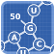RNAblueprint-1.3.2
RNAblueprint library

# Introduction

The RNAblueprint library solves the problem of stochastically sampling RNA/DNA sequences compatible to multiple structural constraints. It only creates sequences that fulfill all base pairs specified in any given input structure. Furthermore, it is possible to specify sequence constraints in IUPAC notation. Solutions are sampled uniformly from the whole solution space, therefore it is guaranteed, that there is no bias towards certain sequences.

The library is written in C++ with SWIG scripting interfaces for Python and Perl. Please cite the software as specified at the bottom of the page!

# Dependencies

Required:

• GNU Automake
• Boost Graph Library
• C++ Standard Library

Optional:

• Boost Programm Options (default: on)
• SWIG for interfaces (default: on)
• Python for interface (default: on)
• Perl for interface (default: on)
• ExtUtils::Embed module for perl interface (default: on)
• Doxygen for documentation
• LaTeX for PDF documentation
• libGMP for multiprecision integers
• Boost Unit Test Framework

# Installation

Just call these commands:

./autogen.sh
./configure
make
make install
In case of a local installation, please do not forget to adopt your path variables such as `PATH`, `LD_LIBRARY_PATH`, `CPLUS_INCLUDE_PATH`, `PYTHONPATH`, `PERL5LIB`

Most important configure options are:

• --prefix Specify an installation path prefix
• --with-boost Specify the installation directory of the boost library
• --disable-program Disable RNAblueprint program compilation
• --disable-swig Disable all SWIG scripting interfaces
• --enable-libGMP Enable the calculation of big numbers with multiprecision

TIP: You might want call `./configure --help` for all install options!

# Interface Examples

## Python example

#!/usr/bin/python
# This script is an example implementation on how to use the Python
# interface. It generates 1000 neighbors of an initially sampled
# random sequence.
import RNAblueprint as rd
# define structures
structures = ['(((((....)))))', '(((....)))....']
# construct dependency graph with these structures
dg = rd.DependencyGraphMT(structures)
# print this sequence
print dg.get_sequence()
# mutate globally for 1000 times and print
for i in range(0, 1000):
dg.sample_clocal()
print dg.get_sequence()
# revert to the previous sequence
dg.revert_sequence();
# print the amount of solutions
print 'Maximal number of solutions: ' + str(dg.number_of_sequences())
# print the amount of connected components
print 'Number of Connected Components: ' + str(dg.number_of_connected_components())
# make a deep copy of the dependency graph
dg1 = rd.DependencyGraphMT(dg)

## Perl example

#!/usr/bin/perl
# This script is an example implementation on how to use the Perl
# interface. It generates 1000 neighbors of an initially sampled
# random sequence.
use RNAblueprint;
# define structures
@structures = ['(((((....)))))', '(((....)))....'];
# construct dependency graph with these structures
\$dg = new RNAblueprint::DependencyGraphMT(@structures);
# print this sequence
print \$dg->get_sequence()."\n";
# mutate globally for 1000 times and print
for(\$i=0; \$i<1000; \$i++) {
\$dg->sample_clocal();
print \$dg->get_sequence()."\n";
# revert to the previous sequence
\$dg->revert_sequence();
}
# print the amount of solutions
print 'Maximal number of solutions: '.\$dg->number_of_sequences()."\n";
# print the amount of connected components
print 'Number of Connected Components: '.\$dg->number_of_connected_components()."\n";
# make a deep copy of the dependency graph
\$dg1 = new RNAblueprint::DependencyGraphMT(\$dg)

## C++ example

// include standard library parts
#include <vector>
#include <string>
#include <iostream>
#include <exception>
extern "C" {
#include "ViennaRNA/fold.h"
#include "ViennaRNA/part_func.h"
}
// include RNAblueprint
#include "RNAblueprint.h"
// typedefs
// functions
float energy_of_structure(std::string& sequence, std::string& structure) {
float energy = energy_of_structure(sequence.c_str(), structure.c_str(), 0);
return energy;
}
float fold(std::string& sequence, std::string& structure) {
char* structure_cstr = new char[sequence.length()+1];
float energy = fold(sequence.c_str(), structure_cstr);
structure = structure_cstr;
delete structure_cstr;
return energy;
}
float pf_fold(std::string& sequence, std::string& structure) {
char* structure_cstr = new char[sequence.size()+1];
float energy = pf_fold(sequence.c_str(), structure_cstr);
structure = structure_cstr;
delete structure_cstr;
return energy;
}
//objective function: 1/3 * eos(1)+eos(2)+eos(3) - 3 * gibbs + 0.5 * 1/3 * (|eos(1)-eos(2)| + |eos(1)-eos(3)| + |eos(2)-eos(3)|)
float objective_function(std::string& sequence, std::vector<std::string>& structures) {
int M = structures.size();
std::vector<float> eos;
for (auto s : structures) {
eos.push_back(energy_of_structure(sequence, s));
}
std::string pf_fold_struct;
float gibbs = pf_fold(sequence, pf_fold_struct);
float objective_difference_part = 0.0;
for (unsigned int i=0; i < eos.size(); i++) {
for (unsigned int j=i+1; j < eos.size(); j++) {
objective_difference_part += abs(eos[i] - eos[j]);
}
}
float eos_sum = 0;
for (int n : eos)
eos_sum += n;
return 1/M * (eos_sum - M * gibbs) + 0.5 * 2/(M * (M-1)) * objective_difference_part;
}
// main program starts here
int main () {
std::vector<std::string> structures;
std::cout << "Input structures in dot-bracket (end with empty line): " << std::endl;
while (true) {
std::string structure;
std::getline(std::cin, structure);
if (structure.empty())
break;
else
structures.push_back(structure);
}
design::DependencyGraph<std::mt19937> * dependency_graph = NULL;
try {
dependency_graph = new design::DependencyGraph<std::mt19937>(structures);
} catch (std::exception& e) {
std::cout << "ERROR: " << e.what() << std::endl;
exit (EXIT_FAILURE);
}
std::cout << "Number of Sequences: " << dependency_graph->number_of_sequences() << std::endl;
//std::cout << "Graph: " << dependency_graph->get_graphml() << std::endl;
for (unsigned int n=0; n<10; n++) {
dependency_graph->sample();
std::string result_sequence = dependency_graph->get_sequence();
float score = objective_function(result_sequence, structures);
for (unsigned int i=0; i<10000; i++) {
dependency_graph->sample_clocal();
std::string current_sequence = dependency_graph->get_sequence();
float this_score = objective_function(current_sequence, structures);
if (this_score < score) {
score = this_score;
result_sequence = current_sequence;
} else {
dependency_graph->revert_sequence();
}
}
std::cout << result_sequence << "\t" << score << std::endl;
}
exit (EXIT_SUCCESS);
}
This file holds the external representation of the DependencyGraph, the main construct for designing ...
Dependency Graph which holds all structural constraints.
Definition: RNAblueprint.h:192
std::string get_sequence()
Get the current RNA sequence as a string.
SolutionSizeType sample_clocal(int min_num_pos, int max_num_pos)
Randomly chooses a connected component with the given size and samples a new sequence for the whole c...
SolutionSizeType number_of_sequences()
Returns the amount of solutions given the dependency graph and sequence constraints.
SolutionSizeType sample()
Resets all bases in the whole dependency graph and samples a new sequence randomly.
bool revert_sequence()
Reverts the sequence to the previous one.

# Testing

Unit tests are available for many functions of the library. Please call `make check` to run these tests!

# How to cite

Stefan Hammer, Birgit Tschiatschek, Christoph Flamm, Ivo L. Hofacker, and Sven Findeiß. “RNAblueprint: Flexible Multiple Target Nucleic Acid Sequence Design.” Bioinformatics, 2017. doi:10.1093/bioinformatics/btx263.